# Texas Go Math Grade 4 Lesson 18.1 Answer Key Fixed and Variable Expenses

Refer to our Texas Go Math Grade 4 Answer Key Pdf to score good marks in the exams. Test yourself by practicing the problems from Texas Go Math Grade 4 Lesson 18.1 Answer Key Fixed and Variable Expenses.

## Texas Go Math Grade 4 Lesson 18.1 Answer Key Fixed and Variable Expenses

Essential Question

What is the difference between fixed and variable expenses?
FIXED EXPENSES:

Fixed expenses are referred to as the cost that does not register a change with an increase or decrease in the number of goods produced by a firm. Fixed costs are those costs that a company should bear irrespective of the levels of production.

Fixed costs are less controllable in nature than variable costs as they are not dependent on the production factors such as volume.

The different examples of fixed costs can be rent, salaries, and property taxes.
VARIABLE EXPENSES:

Variable expenses are referred to as the type of cost that will show variations as per the changes in the levels of production. Depending on the volume of the production in a company, the variable cost increases or decreases.

The various examples of variable costs are the cost of raw materials that are used for production, sales commissions, labour cost, and more.

Money that you need to pay for electricity or phone service are your expenses. Expenses that occur regularly and the amount does not change are fixed expenses. Expenses in which the amount does change based on need or choice are variable expenses.

Unlock the Problem

Erin has some fixed and variable expenses. She pays $23.75 every 12 weeks for the newspaper to be delivered to her home every day. She and her family like to eat out. Monday night, Erin spent$45.78 to eat a meal at Restaurant A. Tuesday night, she spent $58.12 for a meal at Restaurant B. Wednesday night, she spent$37.64 for a meal at Restaurant C.

Example 1 Complete.
Erin’s family is a fixed expense because she pays the same amount and the cost does not change. The money

Erin spends on meals is a ____variable_______ expense because the amount _____varies______ depending on which restaurant she goes to or the type of meal she buys.

Example 2 Find the amount Erin spends on fixed expenses for 24 weeks.

Erin pays $23.75 every 12 weeks for the newspaper In 24 weeks, she pays$23.75 + $23.75 =$ 47.5.

Erin Spends $____47.5________ in fixed expenses for 24 weeks. Answer: 47.5$ Erin spends in fixed expenses.

Math Talk

Mathematical Processes
What other fixed expenses might a person have?
Answer: Here is a list of categories to include in your fixed expenses: Utility bills (cable, cell, electricity, water, etc.) Life / Disability / Extended health (or other) insurance.

Example 3 Solve.
How much does Erin spend on variable expenses?So Erin spent $___________ on variable expenses. Answer: Erin spent$141.54 on variable expenses.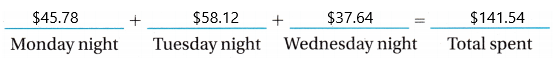Share and Show

Question 1.
Betsy pays a trash service to pick up the household trash once a week. The cost is $23 a month. Trash pick-up is a _____________ expense. In 6 months, the family pays 6 × _____________ = _____________ . Answer: Here trash pick-up is a fixed expense because it is constant for every month that is$23 a month. Now, here the question asked was for one month she has to pay $23 a month. For 6 months how much amount of money she has to pay. In 6 months, the family pays 6×$23=138$. Therefore, the family pays$138 for 6 months.

Question 2.
Mrs. Beyer goes grocery shopping once a week. In one month she spent $99.65,$122.56, $130.45, and$145. What was the total monthly expense? Grocery bills are a variable expense. In a month, Mrs Beyer spent $497.66 on groceries. Answer: Grocery bills are a variable expense. Because for every week amount is changing. Beyer goes shopping weekly once. Here, the question asked about total monthly expenses. We need to add all the amount she spent every week. The total monthly expenses are$99.65+$122.56+$130.45+$145=$497.66.
Therefore, Beyer total expenses are $497.66 Question 3.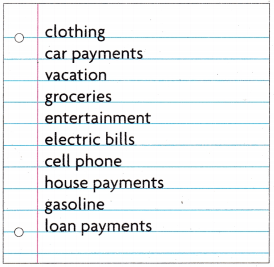Write Math Use the list at the right. Sort the topics into fixed expenses and variable expenses. Choose one and explain why you placed it there.Answer:Explanation: Up to a certain time like years or monthly payments are always constant like car payments, house payments, loan, gas etc. It won’t change their rates. Coming to variable expenses we pay according to the volume we buy a product like clothes, groceries, cell phones etc. Vacations, entertainments, electric bills definitely vary according to the place, situation. so these all are variable expenses. Math Talk Mathematical Processes Explain why some of the topics could be either fixed or variable expenses. Answer: FIXED EXPENSES: Costs that do not change with output means these are operating costs that a business may have that will remain the same, no matter how much they are used. For example, what would be all of the fixed costs of a shoe shop?. Rent, salaries, insurance, broadband. Assume the costs.These all things are fixed in a shoe shop. How much you use is not a matter, if we take broadband, there is no limit to use but every month we have to pay$50 it is the fixed cost. Likewise rent, insurance, salaries.
VARIABLE EXPENSES: Costs that do change with output means these are operating costs that a business may have that will change with use, so the more they are used, the more they will cost. For example, what would be all of the variable costs of a shoe shop?.
Stock, utilities, wages, telephone.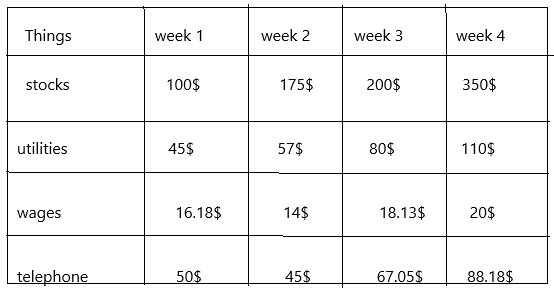Here every week rates change. How much volume do we use according to that we need to pay, for example, take telephone if it is a weekly payment then we pay weekly and how much we use on that week according to that we have to pay. likewise, wages, if he does extra hours the employer will pay the extra amount to the employee.

Problem Solving

Use the numbers in the picture for 4-5.Question 4.
How much has the price of gas increased from the lowest to the highest price shown?
Answer: From the above diagram, we can say the lowest rate and highest rate. The lowest rate is 2.99 and the highest rate is 4.02.
The price of the gas increased was 4.02-2.99=1.03.
Therefore, the 1.03 price increased.

Question 5.
Multi-Step Maya filled her car with gas once at each price shown. Her gas tank holds 15 gallons. Estimate the amount that she spent to fill her tank these four times. Is this a fixed or variable expense?
Given the question:  Maya filled her car with gas once at each price shown in the above diagram are 2.99, 3.99, 4.02, 3.87
The number of tanks she holds=15 gallons.
Now we have to find the amount she spent each time. And moreover, it’s a variable expense because it is changing every time when she fills the gas to her car.
The amount she spent on the first time=15×2.99=44.85$The amount she spent on the second time=15×3.99=59.85$
The amount she spent on the third time=15×4.02=60.3$The amount she spent on the fourth time=15×3.87=58.05$

Question 6.
Apply Mario paid off his car loan this year. His payments were $259 a month for each of the first 8 months. The last payment, in the ninth month, was$125. What was the total Mario paid in car payments this year? Are the payments Mario paid the first 8 months a fixed or variable expense?
Given: Mario paid completely his car loan this year.
The total number of months he paid loan=9
For the first 8 months, he paid 259$each month. So it was a fixed expense because he paid the same amount every month. The total amount he paid in 8 months=259$×8=2072$The amount he paid in 9th month=125$
The total payment Mario paid this year=2072$+125$=2197$Therefore, he paid 2197$ this year.

Question 7.H.O.T Multi-Step Mrs. Xavier buys 1 or 2 books a month for $13.99 each. A magazine subscription costs her$19.99 a year. She buys the Sunday paper for $2.05 each week. Estimate the most she could spend for her reading material in a year. Answer: The number of books Xavier buys each month=2 (given 1 or 2, but take 2). The cost of each book is$13.99.
Xavier spent on books every month=13.99×2=$27.98 For a year she could spend only on books=27.98×12=335.76$
The cost of a subscription for a magazine per year is $19.99 The Sunday paper she buys each week is$2.05
Here the question asked was the amount she could spend on her reading material in a year.  So, we need to find the amount for the Sundays paper for the year.
Sundays paper for the year=2.05×48=98.4$(for a year there are 12 months, in a month there are 4 weeks so 12×4=48 weeks. For 12 months there will be 48 weeks so I multiplied with 48). Now the total amount she could spend=335.76+19.99+98.4=454.15$
Therefore, she spends 454.15$for the year. Question 8. H.O.T. Communicate Wendy’s bakery business has fixed and variable expenses. Her gas and electric bills cost$500 one month. The ingredients cost $700 that month. She pays each of 2 workers$10 an hour to work 40 hours a week. Explain how you can find her total business expenses for a month.
The cost for gas and electric bills for one month=500$The cost of ingredients for a month=700$
The number of workers she has=2
The amount she pays for an hour to each worker is $10. Here 2 workers are there so 10$×2=20$. The total number of hours a week=40 The total amount she was spending every week for 2 workers=40×20=800$
The question asked for total expenses for a month so we need to calculate the salary for the month that could be 800×4=3200$. (In a month there are 4 weeks so I multiplied with 4). Now we have to find, her total business expenses for a month. 500+700+3200=4400$.
Therefore, she spent 4400$every month on her business. Daily Assessment Task Fill in the bubble completely to show your answer. Question 9. Lexi is taking 3 courses at Community College. She pays$835 for each college course. She spends about $45 a week for 20 weeks to drive to school. How much does she spend on fixed expenses? (A)$ 2,505
(B) $2,400 (C)$ 900
(D) $2,700 Answer: Option C is correct.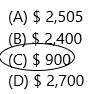Explanation: The number of courses Lexi took at Community College=3 The amount she pays on each course=835$.
The total amount she pays for 3 courses=835×3=2505$. The amount she spends each week=45$
The amount she spends for 20 weeks=45×20=900$The amount she spend on fixed expenses=900$.
Because the charges cannot change but the course costs may change. Here the fixed expenses were only asked. So, the answer is 900$that she is spending every week constantly. Question 10. Every month, Jack’s mother pays the following expenses: the house payment, the club membership, the car payment, and the cost of food. Which is a variable expense? (A) the club membership (B) the house payment (C) the car payment (D) the cost of food Answer: Option D is correct.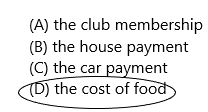Explanation: The variable expense was asked in the given options. The above three options are coming under fixed expenses because they are constant at their rates. But the cost of food will vary because it depends upon the volume we eat that’s why it comes under variable expense. Question 11. Multi-Step Josh has a new cell phone plan. He gets unlimited voice calls and text messages to other devices in his area for$35 a month. Voice calls outside of his area cost $0.10 a minute and text messages cost$0.99 a message. What is Josh’s bill if he uses 10 minutes for calls and makes one text outside his area?
(A) $35.10 (B)$36.10
(C) $35.00 (D)$35.99Explanation:
Given: For unlimited voice calls and text messages within his area is 35$per month. If it’s an outside area then the cost will increase. For voice calls outside the area is 0.10$ and for text messages 0.99$. Now we need to find out Josh’s bill if he uses 10 minutes for calls and makes one text outside his area. That can be written as 35+0.99=35.99$.
josh uses 10 minutes for calls=35 { how much you use in a month that cost could be 35$only it won’t change. It is a fixed expense}. He texts outside the area so he has to pay an extra 0.99$.
Therefore, 35.99$he has to pay. TEXAS Test Prep Question 12. Dara makes bracelets and sells them in her shop. Which is a fixed expense for Dara’s business? (A) the cost of the beads (B) the cost of the string (C) the cost of advertising (D) rent for her shop Answer: Option D is correct.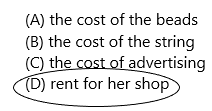Explanation: In Dara’s business, the cost of beads, string, advertising all are coming under variable expenses because they vary according to their volume, quality. But the rent could not change it fixed up to a certain time. So this rent comes under fixed expense. ### Texas Go Math Grade 4 Lesson 18.1 Homework and Practice Answer Key Question 1. Bruce buys 7 cans of dog food a week and a hag of dog food every 4 weeks. Cans of dog food cost$1.29 each.

A bag of dog food costs $67.59. Dog food is a __________ expense. In 4 weeks, Bruce spends 28 × _______ + __________ = __________ for dog food. Answer: Dog food is a fixed expense. It is a constant rate. The number of cans of food Bruce buys each week=7 [for 4 weeks 7×4=28]. The number of bags of dog food he buys=1 He buys dog food every 4 weeks. The cost of each can of dog food is 1.29$
The cost of each bag of dog food=67.59$The amount he spends in a week=7×1.29=9.03$+67.59$×28=2145.36$.
Therefore, he spends 2145.36$on dog food. Question 2.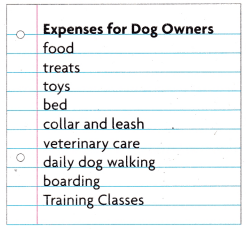Use the list of Expenses for Dog Owners. Sort the expenses into fixed expenses and variable expenses. Choose one expense and explain why you put it where you did.Answer: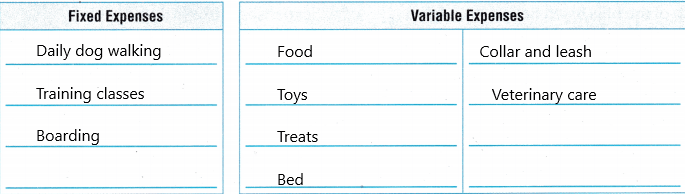Explanation: FIXED EXPENSES: Daily dog walking, training classes, boarding comes under fixed expenses because the cost does not change. we have to pay a fixed amount per month or a year. VARIABLE EXPENSES: Food, we have to pay according to the volume the dog eats. Toys, treats, bed, collar and leash all those things also cost varies according to their size, quality. Veterinary care also the cost varies depending upon the treatment they gave to the dog. Problem Solving Question 3. Gretchen has a cat. She buys a bag of litter every month. Litter costs$38.59 a bag. How much will litter for a year cost? Is the expense fixed or variable?
Answer: 463.08$Explanation: Number of bags of litter she buys every month=1 The cost of a litter of 1 bag=38.59$
The amount she spends in a year on litter=38.59×12=463.08$. Therefore, she spends 463.08$ a year.   { 1year=12 months}.

Question 4
In March, Gretchen bought a brush and a toy for her kitten. The brush cost $6.79. The toy cost$3.88. In April, she bought a cat carrier for $87.68. How much did she spend for these items? Are the expenses fixed or variable? Explain. Answer: 98.35$
Explanation:
The cost of brush=$6.79 The cost of the toy is$3.88
The cost of a cat carrier is $87.68 The total amount she spends on these items=6.79+3.88+87.68=$98.35.
These items are variable expenses because they vary costs depending upon quality and size.
Lesson Check

Question 5.
Mark pays a gardener $100 each week. He bought a fence for the garden and 2 flats of plants. He also bought a shovel and a hoe. Which expense is fixed? (A) gardener (B) plants (C) fence (D) shovel Answer: Option A is correct.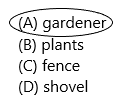Explanation: Mark pays the constant(fixed) amount to the gardener. How much the gardener works does not matter, the gardener will be paid by Mark$100 every week. So it is a fixed expense.

Question 6.
Leslie rides the bus to work and back 5 days a week. The bus costs $1.25 each way. Sometimes she buys a newspaper to read on the bus. The newspaper costs$0.75 a day. How much does she spend for fixed expenses every week?
(A) $6.25 (B)$12.50
(C) $18.75 (D)$10.00The number of days she will take to come back=5
The cost for each way=$1.25 For 5 days=1.25*5=6.25 The cost of a newspaper=$0.75
For 5 days=0.75*5=3.75
The amount she spends on fixed expenses every week=6.25+3.75=10.

Question 7.
Every month, Wesley writes checks to pay each of the bills listed below. Which is a variable expense?
(A) rent
(B) cable television
(C) electricity
(D) gym membershipExplanation:
Electricity comes under variable expense because it depends upon you much power we use every day or every month. If we consume more power then more amount we have to pay. If we consume less then we will pay less.

Question 8.
Which of Victoria’s monthly car expenses is fixed?
(A) car payment
(B) gas
(C) bridge tolls
(D) parking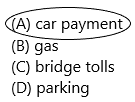Explanation:
Car payment is fixed because if we kept instalments to pay then we have to pay a certain amount monthly which is fixed. All other options are coming under variable expenses because their cost depends upon the places. So definitely they will vary.

Question 9.
Multi-Step A store owner pays $1,200 each month for rent. She pays. the clerk who works in the store$15 an hour. The clerk works 60 hours each month. What is the total of the monthly fixed expenses?
(A) $2,100 (B)$1,290
(C) $1,800 (D)$3,00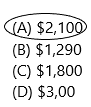The rent she pays every month=$1200 She pays the clerk per hour=$15
The total amount she spends monthly on fixed expenses=1200+900=$2100 Question 10. Multi-Step Jenny spends$2.20 for a school lunch every day. She also buys a muffin at the bakery every day on her way home from school. The muffin costs $1.25. What are Jenny’s fixed expenses for a 5-day school week? (A)$15.05
(B) $15.75 (C)$22.25
(D) $17.25 Answer: Option D is correct.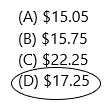The cost Jenny spends on lunch every day=$2.20
The cost of muffin she buys every day=$1.25 For 5 days=1.25*5=6.25 The total cost she spends for 5 days=11+6.25=$17.25.